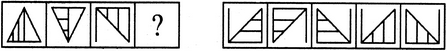# Non Verbal Reasoning - Analogy - Discussion

### Discussion :: Analogy - Section 1 (Q.No.23)

Each of the following questions consists of two sets of figures. Figures A, B, C and D constitute the Problem Set while figures 1, 2, 3, 4 and 5 constitute the Answer Set. There is a definite relationship between figures A and B. Establish a similar relationship between figures C and D by selecting a suitable figure from the Answer Set that would replace the question mark (?) in fig. (D).

23.

Select a suitable figure from the Answer Figures that would replace the question mark (?).

Problem Figures:                            Answer Figures:(A)     (B)      (C)     (D)                  (1)      (2)      (3)      (4)      (5)

 [A]. 1 [B]. 2 [C]. 3 [D]. 4 [E]. 5

Explanation:

The figure gets vertically inverted and the two vertical lines inside the figure get rotated through 90o.

 Sandeep said: (May 31, 2014) This can be interpreted as, the figure gets rotates 180 CW, and the vertical lines inside the figure get rotated through 90. So answer could be C.

 Himanshu Jain said: (Sep 4, 2020) Answer should be D.

 Ravi said: (Dec 16, 2020) The right Answer will be C.

 Shruthi said: (Dec 31, 2020) It will be C if you compare the lines in the figures as horizontal and vertical.

 Himesh said: (Aug 8, 2021) The Right answer should be C.

 Asiya said: (Sep 15, 2021) Answer should be B.

 Asiya said: (Sep 15, 2021) Answer should be B.

 Sejal J said: (Oct 24, 2021) The answer should be C.

 Kamal said: (Jul 30, 2022) I think it should be option C.